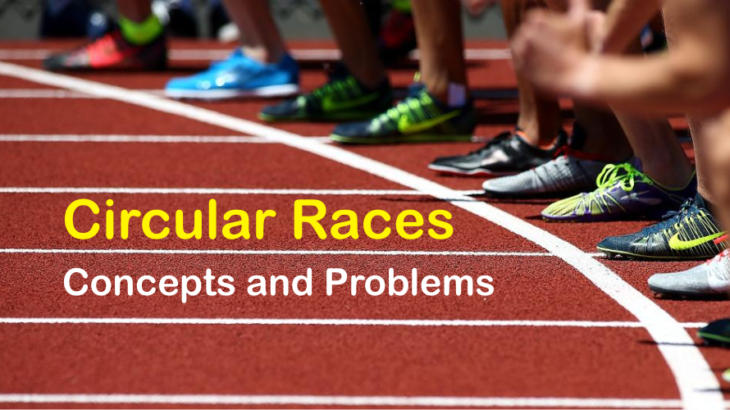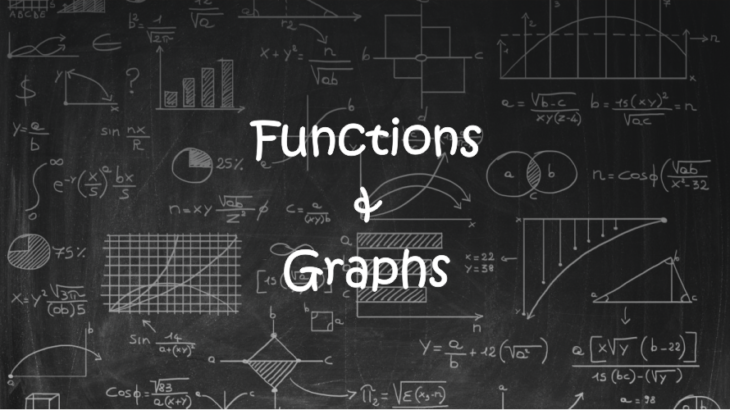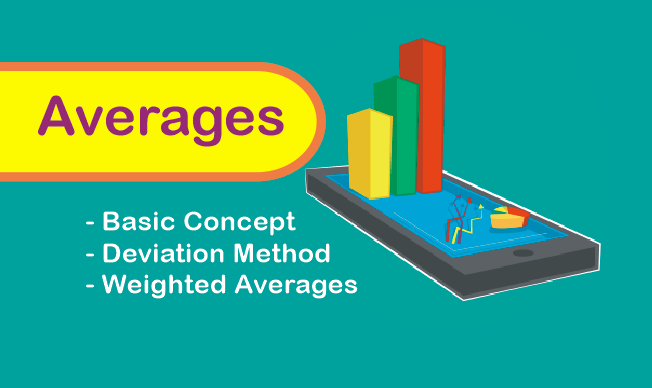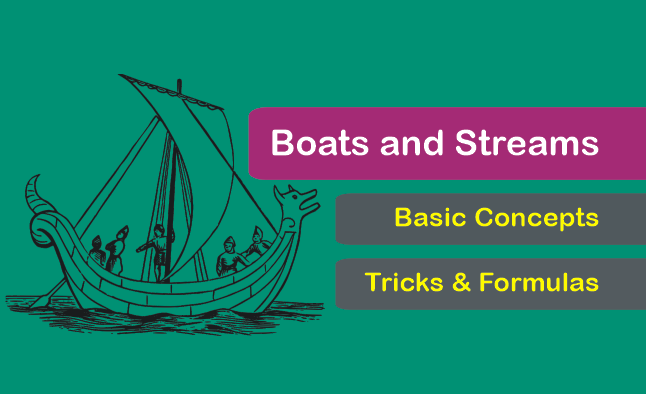Circular Races: Concepts Tricks and Problems for CAT ExamCircular races problems have been both exciting and tantalizing to students who prepare for the CAT exam. Often the problems on circular track revolve around finding the number of distinct meeting points or the time required by the runners to meet for the first time at the starting point. Concepts and tricks require to solve […]

Geometry Formulas for Area of the Triangle for CAT Exam

There are many formulas to calculate the area of a triangle. Some formulas are very frequently used and some are used specifically for certain types of questions. A student should not only remember those formulas but also know where to apply them. In this article will discuss all the formulas for finding the area of a […]

CAT Geometry Concepts and Questions: Triangles

Fundamental concepts of Triangles Under the geometry section of various competitive exams like CAT, the most critical topic within geometry is Triangle. Many questions from triangle are based on the basic concepts like the understanding of sides and angles and a few elementary theorems on triangles. It is interesting to note that the questions can be […]

Basic Concepts of Functions and Graphs for CAT ExamsIn CAT Exam, certain kind of questions from algebra requires higher levels of algebraic concepts. However, such questions can also be easily solved by using alternate approaches from graphs. Below are a few questions from CAT and other exams, which would be very difficult to crack if we try solving them using the concepts from […]

Concept of average and short tricks [Method of Deviation] for CAT ExamConcept of averages for CAT An average is yet another essential concept for the CAT and other competitive exams. Most of us have a fair understanding of the average or mean. However, the questions from average which appear in competitive exams are tricky and often calculation intensive if we apply the standard approach. In this article, […]

Boats and Streams: Formulas Concepts and Questions for CAT ExamBoats and Streams is an essential topic for many competitive exams. Many varieties of questions can be framed from this area. We use the fundamental concepts of time speed and distance only to solve elementary questions on Boats and Streams. However, some types of questions are tricky and take lots of time to solve by […]

Profit and Loss: Basic Concepts and Shortcuts for CAT ExamProfit and loss based problems in CAT can easily be solved by applying the fundamental concepts of percentages. The following basic terms are widely used while solving problems on profit and loss. Hence, a clear understanding of each of these terms is essential. Terminologies in Profit and Loss Cost Price (C.P): The price at which an […]